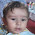# Difference between CHAID and CART

Classification and Regression Trees (CART)

Regression Tree : The outcome (dependent) variable is a continuous variable and predictor (independent) variables can be continuous or categorical variables (binary). It creates binary split.

Algorithm of Regression Tree:  Least-Squared Deviation or Least Absolute Deviation

The impurity of a node is measured by the Least-Squared Deviation (LSD), which is simply the within variance for the node.

Classification Tree : The outcome (dependent) variable is a categorical variable (binary) and predictor (independent) variables can be continuous or categorical variables (binary). It creates binary split.

Note : If the dependent variable has more than 2 categories, then C4.5 algorithm or conditional inference tree algorithm should be used.

Algorithm of Classification Tree: Gini Index

Gini Index measures impurity in node. It varies between 0 and (1-1/n) where n is the number of categories in a dependent variable.

Process :
1. Rules based on variables' values are selected to get the best split to differentiate observations based on the dependent variable
2. Once a rule is selected and splits a node into two, the same process is applied to each "child" node (i.e. it is a recursive procedure)
3. Splitting stops when CART detects no further gain can be made, or some pre-set stopping rules are met. (Alternatively, the data are split as much as possible and then the tree is later pruned.

CHAID

CHAID stands for Chi-square Automated Interaction Detection.

The outcome (dependent) variable can be continuous and categorical. But, predictor (independent) variables are categorical variables only (can be more than 2 categories). It can create multiple splits (more than 2).

When independent variables are continuous, they need to be transformed into categorical variables (bins/groups) before using CHAID.

Algorithm :

If dependent variable is categorical, Chi-Square test determines the best next split at each step.

If dependent variable is continuous, F test determines the best next split at each step.

Process :

Cycle through the predictors to determine for each predictor the pair of (predictor) categories that is least significantly different with respect to the dependent variable; for classification problems (where the dependent variable is categorical as well), it will compute a Chi-square test (Pearson Chi-square); for regression problems (where the dependent variable is continuous), F tests. If the respective test for a given pair of predictor categories is not statistically significant as defined by an alpha-to-merge value, then it will merge the respective predictor categories and repeat this step (i.e., find the next pair of categories, which now may include previously merged categories). If the statistical significance for the respective pair of predictor categories is significant (less than the respective alpha-to-merge value), then (optionally) it will compute a Bonferroni adjusted p-value for the set of categories for the respective predictor.

Selecting the split variable. The next step is to choose the split the predictor variable with the smallest adjusted p-value, i.e., the predictor variable that will yield the most significant split; if the smallest (Bonferroni) adjusted p-value for any predictor is greater than some alpha-to-split value, then no further splits will be performed, and the respective node is a terminal node.

Continue this process until no further splits can be performed (given the alpha-to-merge and alpha-to-split values). (Source : Statsoft)Comparison of CHAID and CART

How CHAID is better than CART ?

1. CHAID uses multiway splits by default (multiway splits means that the current node is splitted into more than two nodes). Whereas, CART does binary splits (each node is split into two daughter nodes) by default.
2. CHAID prevents overfitting problem. A node is only split if a significance criterion is fulfilled.Related PostsDeepanshu founded ListenData with a simple objective - Make analytics easy to understand and follow. He has over 8 years of experience in data science. During his tenure, he has worked with global clients in various domains like Banking, Insurance, Telecom and Human Resource.

4 Responses to "Difference between CHAID and CART"
1.Beautifully Explained... Thanks a lot!! Clears my confusion.

1.Glad you found it useful. Cheers!

2.Never understood Cart and Chaid before this... very clearly explained. Thanks

3.Very good explanation, thank you so much sir.

Next → ← Prev
Love this Post? Spread the Word!
Share